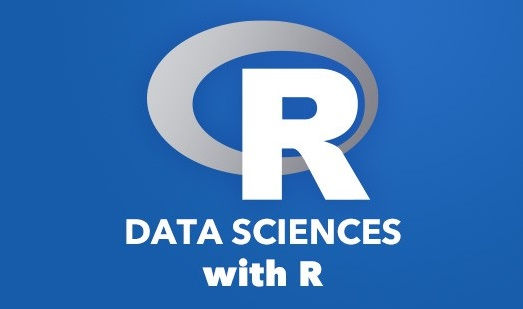top of page
Search

# Data science With R

Updated: Jan 27, 2022Data science is an interdisciplinary field that allows us to extract meaningful information, knowledge and insights from structured and unstructured data. R is the open source programming language and free software environment for data manipulation, statistical analysis and graphics representation. It is mainly used for developing statistical software and data analysis.

Introduction to R

R is the programming language which is mainly used for statistical analysis, exploratory data analysis and graphical representation. R is completely free and open source and an active community member. It is compatible across all platforms (Linux, Windows and Max) and also it has an extensive library of packages for machine learning. It can be easily integrated with software like tableau, SQL server etc.

Data science concept

Business problem : Understand your business problem first and figure out what your machine learning model will do for you like predict something or create etc. It is important to understand the problem to solve them and we can use the right algorithm to tackle the problem.

Data preparation

• Data collection : It is the process of gathering data and combining the data from multiple sources.

• Data cleaning : Data cleaning is the process of detecting and correcting the missing or incorrect data from the record set, table or database. it refers to identifying null, incorrect or irrelevant parts of the data and then replacing, modifying, or deleting the dirty or coarse data

• Transformation : Data transformation is the process converting the raw data into a format that can be used or more suitable for model building.

Exploratory Data Analysis (EDA)

Exploratory data analysis is the process of analyzing the data and presenting the result in simple format for easy understanding.

Algorithm for Data science

Data modeling : Identify the model that best fits the business requirements. Train the model on the training data set and test.

Supervised

• Random forest

• Naive bayes

• KNN

• Decision Tree

• Logistic regression

• Support vector machine

Unsupervised

• K-means clustering

• Hierarchical clustering

• Gaussian mixture model

Data Visualization in R

Data visualization is the graphical representation of data and information, by using visual elements such as charts, maps and graphs etc.

• Aesthetics Mappings

• Single Variable Plots

• Two-Variable Plots

• Facets, Layering, and Coordinate System

ggplot2 -This package is used to create a chart and visualization such as density plot, violin plot, blox plot etc.

Data science packages in R

Data manipulation in R

Following techniques are mostly used for data manipulation in R.

• Tidy Data - Tidy datasets provide a standardized way to link the structure of a dataset with its semantics

• Dplyr : Dplyr packages aim to provide a function for each basic verb of data manipulation.

• filter() - It is used to extract a subset of rows from a data frame based on logical conditions.

• Arrange() - The Arrange() function is used to reorder rows of a data frame

• select() - The select() function creates a new subset of the columns of a data frame, using a flexible notation.

• rename( )- It is used to change the name of an individual variable.

• Mutate() - It adds new variables/columns or transforms existing variables.

• group_by() -It is used to group the data frame by single column or multiple column with functions such as count, maximum, minimum, mean etc.

• summarise() : This function is used to generate statistics summaries of different variables in the data frame.

• The Pipe - %>% Pipe operators are used to connect multiple action verbs together into a pipeline.

• String Manipulation - it is the process of handling and analyzing the strings.

• Web Scraping - It is a technique to extract the data from the web, convert it into structured format which can easily be accessed and used.

DATA TYPES/STRUCTURES IN R

• Vectors - In R programming language, c() function is used to create vectors of objects by concatenating things together.

• Matrices - Matrices are vectors with a dimension attribute. The dimension attribute is itself an integer vector of length, number of rows and number of columns.

• Lists - It is a special type of vector that contains elements of different classes and it is a very important data type in R programming language and it is explicitly created using the list() function, which takes an arbitrary number of arguments.

• Data Frames - It is used to store the tabular data in R.

• Operators - In R, Operator is used to perform the specific mathematical and logical operations

• Loops - In R language, loops is a control statement that allows multiple execution statements or a set of statements.

• Functions - In R programming language, A function allows to create an interface to the code, that is explicitly specified with a set of parameters and this interface provides the abstraction of code to potential users.

### Data science project in R

Here we will show you by implementation data science project in R

Import the data set

code :

```# import dataset

output :Extract only features column

code :

`data = data[,3:5]`Split the dataset

code:

```library(caTools)
set.seed(123)
data_split = sample.split(data\$Purchased, SplitRatio = 0.75)
train_set = subset(data, data_split == TRUE)
test_set = subset(data, data_split == FALSE)```

Ouptut :Feature scaling

code :

```# feature scalling
train_set[,1:2] = scale(train_set[,1:2])
test_set[,1:2] = scale(test_set[,1:2])```### Codersarts provide:

• R prograaming Assignment help

• R programming Error Resolving Help

• Mentorship in R programming from Experts

• R programming Development Project

If you are looking for any kind of Help in R programming Contact us

See All
bottom of page